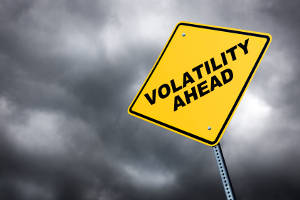### What will the FTSE’s Low be in 2014?It’s that time of year when everyone is getting their predictions in for 2014.

Will equities enjoy another bumper year or will returns be more modest … or will they be negative ?

Not surprisingly given we are in the midst of a bull-market a lot of the talk is of how much higher equities could go in 2014, my expectation is also that there will be positive returns to equities, but I’m much more interested in thinking about the volatility – and specifically what that tells about the likely low point that the index will experience in the next year.

I’m not a massive fan of predictions, I prefer to look at what the information currently available in the market is telling us. And when it comes to the subject of the likely highs and lows over the next 12 months, it tells us quite a lot.

As most readers will no doubt appreciate, the likely  ranges for the level of the FTSE 100 – or any other equity index –  over the next 12 months will be driven by the volatility. But exactly how can one calculate what these ranges should be?

If we make some simplifying assumptions about the behaviour of the level of the FTSE 100 index, then it turns out that the probability of the index hitting (or exceeding) any given level during the year is incredibly simple to calculate, using the elegantly simple refelction principle* this probability is equal to twice the probability of the same level being hit or exceeded at the end of the year, which in turn is easy to calculate using relatively straightforward option maths.

ok ok, enough theory – show me some examples!

Easy enough – so the FTSE 100 started 2014 around the 6750 mark. As always, volatility needs to be estimated, and while this can of course increase or decrease during the year, the price of options on the FTSE 100 gives us an estimate, or implied, level for the volatility. This implied volatility tells us something about the market’s expectations for the fluctuations in the FTSE 100 index next year.

I’ve used the full information provided by prices of all available options, or the full “volatility smile” as it is known. This allows for some possibility that volatility could increase as the market falls.

FTSE 100 start value = 6750

Probability of finishing the year below 5950 = 25% [calculated as the delta of a 1-year 5950 strike put option]

Probability of falling below 5950 at any point during the year = 50% [ ie, 2 x 25% ], ie the median expected index low for the year is 5,950.

this calculation uses a volatility of around 17%, which is the current market-implied volatility for an option with a strike of 5,950.

By the same calculations our median expectation for the high point during the year is around 7200 (ie, the level at which there is a 50% probability of hitting of exceeding during the year). This would represent a substantial new high for the index, in fact starting from a level of 6,750, the probability of making a new high (above 6,950, the level which was reached back in 1999) is around 68%, based on the implied volatility in the market at the start of January.

Now, to calculate these I’ve clearly estimated some important parameters, including the average drift of the Brownian motion which drives the index level. The table below shows how the expected high and low would change at different levels of volatility.

 Vol (%) >> 15% 20% 25% Drift >> 0% 6000 5900 5750 2% 6150 6000 5850 4% 6250 6100 5975

We can repeat the same calculations for other equity indices around the world, given the levels of volatility implied in those markets.

 Implied vol Expected Low ESTOXX 20.0% 2700 S&P 500 18.8% 1625 Nikkei 24.5% 14150

That’s interesting, but enough examples, what are you trying to tell me?

1.Equities are pretty volatile, and fixating on predictions of where they will be in a year’s time ignores what we can tell about the fluctuations along the way – which are large

2. While we can easily talk about a volatility of say 10%, 15% or 20%, its often easy to forget what this actually means in a world where equities are increasing in value each year. What it means is that its quite normal for us to get pretty big swings in value intra-year – for example a rally in the FTSE to over 7,200 followed by a fall to 6,000 would not be inconsistent with historical levels of volatility. Indeed this sort of move should be expected.

3. the key estimate that determines the extent of fluctuations next year is the volatility, which we can estimate using historical data but can’t pin down exactly, also it can change during the year. The real risk is that risk increases

4. Note: I am not making a prediction that equity returns will be negative over 2014 as  whole, my point is that we can probably say more about the fluctuations we’ll see during the year, than we can about the end result.

* The reflection principle reasons, quite intuitively that for any path (of an equ ity stock or index) that hits a given line, we can visualize both the original path continued, and a “reflected” path which follows a reflection of the true path. Ultimately one of these two will finish the time-period above the line, and one will finish below. Given each has equal probability we can then reason that the probability of hitting the line at any time during the period is equal to twice the probability that we end the period above the line. There is a “proper” mathematical explanation of this too, which can be found here.

Posted in GK